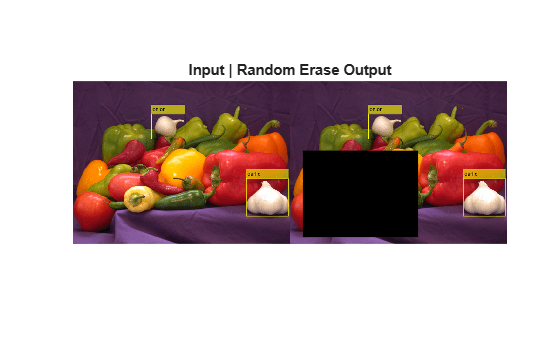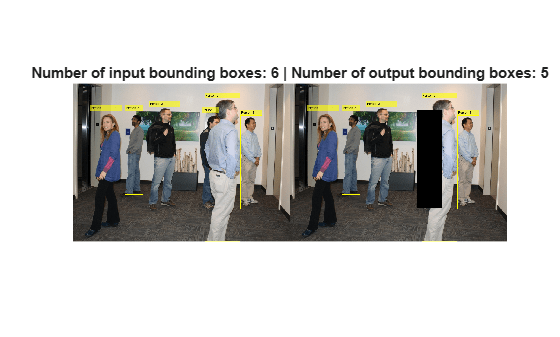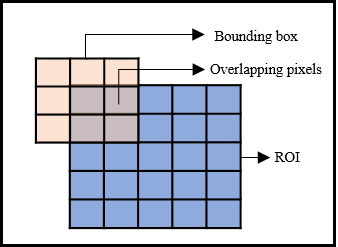# bboxerase

Remove bounding boxes

Since R2021a

## Syntax

``bboxB = bboxerase(bboxA,window)``
``[bboxB,indices] = bboxerase(bboxA,window)``
``___ = bboxerase(___,EraseThreshold=threshold)``

## Description

example

````bboxB = bboxerase(bboxA,window)` removes bounding boxes in the input `bboxA` that lie within a region of interest (ROI) specified by `window`. The output is the set of bounding boxes retained from the input `bboxA`. This function supports 2-D and 3-D bounding boxes. NoteTo perform random erase or cutout data augmentation, use `bboxerase` along with the `imerase` function. ```
````[bboxB,indices] = bboxerase(bboxA,window)` also returns the indices of the bounding boxes retained from the input set of bounding boxes `bboxIn`.```

example

````___ = bboxerase(___,EraseThreshold=threshold)` additionally specifies the threshold for the amount of overlap between a bounding box region and the specified ROI. A bounding box is removed if the overlap between the bounding box region and the ROI is equal to or greater than the specified threshold.```

## Examples

collapse all

`I = imread('peppers.png');`

Define bounding boxes and labels.

```bboxA = [410 230 100 90; 186 78 80 60]; labelsA = ["garlic";"onion"];```

Find the size of the input image

`inputSize = size(I);`

To randomly select a region of interest (ROI), specify a range for the scale and the aspect ratio of the ROI. The scale value for the ROI is set to lie in the range 0.2 and 0.3. Similarly, the minimum value for the aspect ratio is set to lie in the range 1:10 and the maximum value for the aspect ratio is set to lie in the range 30:100.

```scale = [0.2,0.3]; dimensionRatio = [1,10;30,100];```

Select the ROI by using the `randomWindow2d` function.

`window = randomWindow2d(inputSize,'Scale',scale,'DimensionRatio',dimensionRatio);`

Remove the pixels and the bounding boxes that lie within the randomly selected ROI.

```J = imerase(I,window); [bboxB,indices] = bboxerase(bboxA,window); labelsB = labelsA(indices);```

Display the original and augmented image.

```annotatedI = insertObjectAnnotation(I,'Rectangle',bboxA,labelsA); annotatedJ = insertObjectAnnotation(J,'Rectangle',bboxB,labelsB); figure montage({annotatedI,annotatedJ}) title(['Input | Random Erase Output'])````I = imread('visionteam1.jpg');`

Define bounding boxes and labels.

```bboxA = [64 101 117 440; 193 101 67 309; 282 86 114 375; 618 118 79 345; 486 55 131 528; 475 109 66 361]; labelsA = ["Person 1";"Person 2";"Person 3";"Person 4";"Person 5";"Person 6"];```

Specify a rectangular region of interest.

`window = [470 100 90 360];`

Erase the pixels that lie within the rectangular region by using `imerase` function.

`J = imerase(I,window);`

Erase the bounding boxes that lie within the rectangular region. Set the erase threshold value to 0.7. The `bboxerase` function returns the retained bounding boxes and the corresponding indices.

`[bboxB,indices] = bboxerase(bboxA,window,'EraseThreshold',0.7);`

Read the class labels corresponding to the retained bounding boxes.

`labelsB = labelsA(indices);`

Display the results.

```figure I = insertObjectAnnotation(I,'Rectangle',bboxA,labelsA); J = insertObjectAnnotation(J,'Rectangle',bboxB,labelsB); imshowpair(I,J,'montage') title(['Number of input bounding boxes: ', num2str(length(labelsA)), ' | ', 'Number of output bounding boxes: ', num2str(length(labelsB))])```## Input Arguments

collapse all

Input set of bounding boxes, specified as M-by-4 matrix. M is the number of bounding boxes. Each row in the matrix of form [xmin ymin width height]. [xmin ymin] are the top left coordinates of the bounding box. width and height are the width and the height of the bounding box respectively.

Data Types: `single` | `double` | `int8` | `int16` | `int32` | `int64` | `uint8` | `uint16` | `uint32` | `uint64`

Region of interest, specified as one of these values:

• 4 -element vector of form [x y width height]. [x y] are the top left coordinates of the ROI. width and height are the width and the height of the ROI respectively.

• `Rectangle` object.

Data Types: `single` | `double` | `int8` | `int16` | `int32` | `int64` | `uint8` | `uint16` | `uint32` | `uint64`

Erase threshold, specified as a positive scalar less than or equal to 1. The erase threshold sets the criteria for removing a bounding box from the input set `bboxA`. The value is the ratio of number of pixels in a bounding box that lie inside the ROI (overlapping pixels) to the total number of pixels in the bounding box.`$threshold\text{\hspace{0.17em}}=\text{\hspace{0.17em}}\frac{Number\text{\hspace{0.17em}}of\text{\hspace{0.17em}}overlapping\text{\hspace{0.17em}}pixels}{Total\text{\hspace{0.17em}}number\text{\hspace{0.17em}}of\text{\hspace{0.17em}}pixels\text{\hspace{0.17em}}in\text{\hspace{0.17em}}bounding\text{\hspace{0.17em}}box}$`

The default value is 0.8. This implies that, a bounding box is removed if 80 % of the pixels in the bounding box lie within the specified ROI.

Data Types: `single` | `double` | `int8` | `int16` | `int32` | `int64` | `uint8` | `uint16` | `uint32` | `uint64`

## Output Arguments

collapse all

Output bounding boxes retained from the input, returned as a N-by-4 matrix. N is the number of bounding boxes retained from the input. Each row of the matrix defines one bounding box of the same type as the input `bboxA`. The value of N is always less than or equal to M, the number of input bounding boxes.

Data Types: `single` | `double` | `int8` | `int16` | `int32` | `int64` | `uint8` | `uint16` | `uint32` | `uint64`

Indices of retained bounding boxes, returned as a vector of integers. The indices indicate which bounding boxes in the input, `bboxA`, are retained and returned at the output.

## Version History

Introduced in R2021a